﻿ Space EquationsInternational Journal of Theoretical and Mathematical Physics

p-ISSN: 2167-6844    e-ISSN: 2167-6852

2019;  9(2): 41-44

doi:10.5923/j.ijtmp.20190902.03### Space Equations

Prince Jessii

Trasker Institute of Scientific Research, 266 Main Rd, VV Mohalla, India

Correspondence to: Prince Jessii, Trasker Institute of Scientific Research, 266 Main Rd, VV Mohalla, India.
 Email: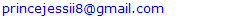Abstract

Trying to observe the reason behind the differences in the nature of space that exists on earth and on the outer space led to the path were space and gravity meets. This paper presents a theory which comprises of an already existing effect that has helped to determine the following;• Space constant.• The Relationship between Space and Gravity.• Formulation of Space Equations.• Verification of the value of acceleration due to gravity, mass, radius of most planetary bodies.• The Fate of the existence of parallel universes.

Keywords: Space, Gravity, Planet

Cite this paper: Prince Jessii, Space Equations, International Journal of Theoretical and Mathematical Physics, Vol. 9 No. 2, 2019, pp. 41-44. doi: 10.5923/j.ijtmp.20190902.03.

### 1. Introduction

We can proceed by defining space as a boundless three dimensional extent in which objects and events have relative position and direction. Space was lately addressed with time to be part of a boundless four-dimensional continuum known as space-time or space-time fabric. However, space is occupied by different kinds of matter with different masses. On the outer space, the space around such is known to be of a fabric nature of high magnitude in which objects don’t fall or move downwards, the objects of masses are rather found to be distorting the space fabric instead of moving downwards. Besides, if we ask the question why doesn’t the space fabric exist on earth, biologically we could say, in creation, the idea was to allow the planet earth to harbor living things that will be able to move and carry out their activities freely, the otherwise will not be suitable. However, scientifically there should be a cause of this mystery which we will find out at the end of this paper.

### 2. Discussion

#### 2.1. The Containment of Space

Space at its strongest is in a form of a fabric which can be reduced to a very free space as its lowest form. Space as a fabric is the original form of space that holds the planetary bodies and prevent them from moving or falling downwards.
Using a known example, when a rocket takes off and reaches the outer space, initially, the space inside the rocket is a free space while on earth, but while on the outer space, it’s a stiff one [fabric]. So it’s true, we can say that space cannot be contained, rather as an object moves, it moves through the space around it.
Meanwhile, if we say space cannot be contained, why are there planets which contain another form of space in an environment of space fabric [outer space].

#### 2.2. The Anti-Space Effect

We can proceed from here. Space fabric can be distorted by the action of mass which can result to a curvature. All planetary bodies where created in this environment [space fabric], therefore all planetary bodies contained space fabric at the point of creation. Once any of these planetary bodies was found to distort the space fabric, there came up this effect which is the only reason any form of space will exists with another form on the same enclosure.
Definition: The action of a body of mass on the original form of space [space fabric] results in a distortion which brings about an effect that acts on the space inside the body of mass.
This is called the Anti - space Effect, which depends on the space constant, the mass of the body and the radius of body. The anti-space effect will be addressed as ANSP effect as we proceed.
Space Constant: The space constant which can be addressed as an invisible space fabric that exists on the outer space.
The Mass: A body of mass which is observed to be distorting the space fabric. The higher the mass of the body, the more extent to which the fabric is stretched downwards [distortion], which leads to a higher ANSP effect.
The Radius of the body: The ANSP effect reaches to a particular distance across the body.

#### 2.3. An Illustration of the ANSP Effect

In this illustration, we will make use of a body of low mass with radius, a body of a moderate mass with radius and a body of high mass with radius.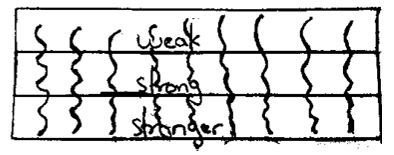Figure 1
Figure 1 shows the nature of space fabric, inside is divided into segments which shows the different levels the ANSP effect would be as the body of mass distorts the fabric.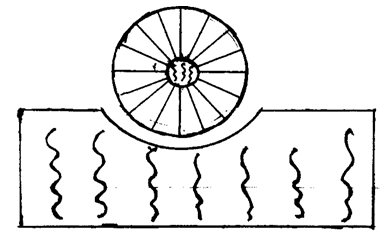Figure 2
Figure 2 represents a body of low mass distorting the space fabric, inside the body of mass exists two different nature of space.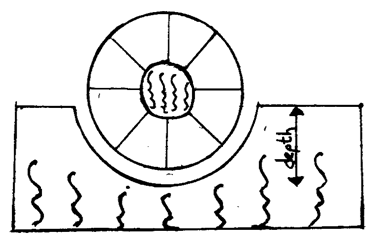Figure 3
Figure 3 represents a body of moderate mass distorting a space fabric which results in differences in nature of space inside it. It differs from the previous diagram in a way that the space on the outer sphere becomes free, this is due to a high ANSP effect resulting from a deeper distortion caused by the mass.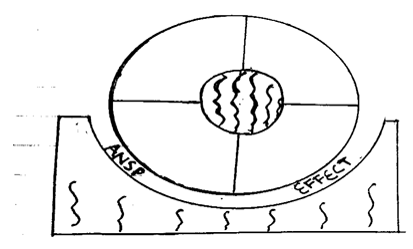Figure 4
Figure 4 represents a body of large mass distorting a space fabric resulting to a difference in nature of space inside the body of mass. The space on the outer sphere becomes very free. Thus, the ANSP effect in these diagrams are known to exists within the curvature which is illustrated above.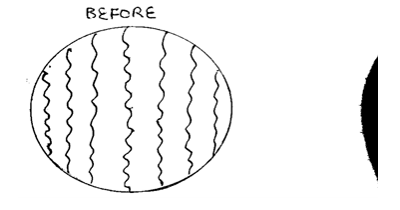Figure 5
Using a planet on the outer space as an example, figure 5 is a planet which contained space fabric while forming at the point of creation.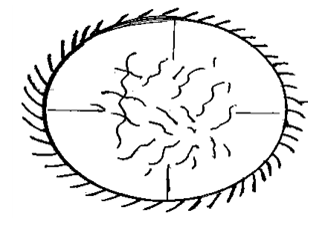Figure 6
Figure 6 shows the action of ANSP effect on the planet. The effect spreads uniformly and surrounds the surface of the planet, it reduces the magnitude of the space fabric inside it gradually around the planet to a particular distance [depending on the size of the planet] thereby bringing a different nature of the space on the affected area.
The nature of the space on the affected area depends the rate of the ANSP effect [low or high].
The higher the ANSP effect rate, the higher the rate of reduction of the space fabric.
On the other hand, the rate of the ANSP effect depends on how deep the planet is distorting a space fabric, and the depth of the distortion depends on the mass of the planet.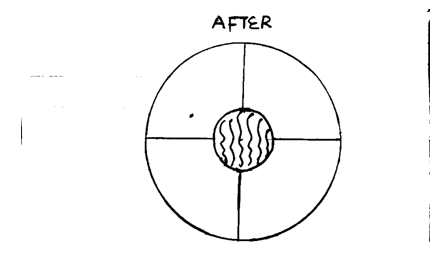Figure 7
Figure 7 shows how free the space on a big size planet with mass will be after been affected by ANSP effect of a high rate. The space fabric which was contained inside initially is been reduced to a very free space around it and out to a particular distance were it reaches.
Since the effect clears it to a particular distance, the size of the planet will determine how large the area of the space fabric around the center would be. However, the space fabric in which the ANSP effect could not reach is located at the center of the body, which can be described as the core of a planet, it is responsible for holding the inner parts of the planet together.

#### 2.4. Where Space and Gravity Meet

Using our solar system, all 8 planets are affected by the distortion caused by the sun on space fabric. Lately, gravity was described to be a consequence of curvature and not a force. A consequence of curvature in the sense that the curvature makes all eight planets to have an attraction to the sun. This curvature is as a result of the distortion caused by the sun, distortion is associated with depth and curvature is associated with the width, but they are of the same cause, which means distortion causes curvature. However, on the outer space, the moon is on the earth’s curvature and both with the eight planets are on the sun’s curvature. Once a planet is on the sun’s curvature, it is inside the zone of the ANSP effect of the sun which will cause an attraction by the sun on the planet. On the other hand, the planet has its own curvature with its ANSP effect that also attracts, which means, between the sun and the planet, there exists a point of repulsion from both bodies thereby allowing the planet not to fall to the sun which has a higher mass, this situation also exists between planets and their moon.
However, following one path[space], it is found it to be an ANSP effect, but following the other path, it is found to be gravity. However, these two intersect at point where we conclude that;
Gravity is an anti-space fabric effect which exist from the distortion caused by the action of mass on a space fabric.
To proceed, our Anti-space effect is now replaced with gravity. Space and Gravity always work together to draw objects of mass towards the center of the Earth, which makes space the base of acceleration due to gravity. This means that gravity [ANSP effect] which is on the outside [outer space] reduces the magnitude of the space fabric which was originally on the inside of the distorting mass [earth] to a free space, which makes objects to stay freely at its position.
In summary, we observe that, in a body of large mass [Earth] that is affected by a high gravity which results to a very free space, objects tend to fall back to the center of the body, but it falls back fast to the ground [the rate depends on the nature of space].
Secondly, in a body of small mass [Moon] that is affected by a lower gravity but has a less free space, objects fall back to the center of the body, but it falls back slowly [the rate depends on the nature of space].
In all, we can say that if we could to go through [200-300 feet] from the ground, we will feel a different nature of the space around us as we approach the center of the earth.

### 3. Methods

#### 3.1. Space Equations

 Table 1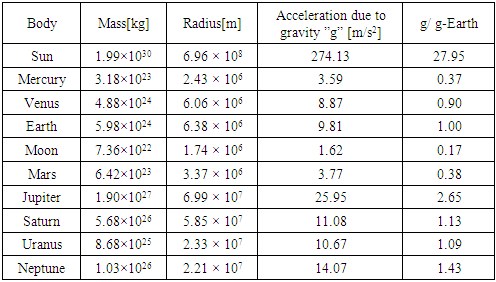Table 1 shows values of acceleration due to gravity(g), mass(M), and radius(r) for planets in our solar system.
Using the values of g, M and r for Earth, we can determine the space constant
We can recall that the ANSP effect involves four parameters
The mass of the body, M
The space fabric which is the space constant, S
The radius of the body, r
The space contained by the body,
An equation which comprises of these four parameters is derived and is given below as;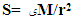(1)
Which also means the space contained by body, ﯼ is given by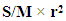(2)
g is found to be the reciprocal of ﯼ, which is 1/
Using the value of g on earth which is 9.8m/s2
The value of ﯼ on earth will be 1/9.8 which is 0.102.
Putting the value of M, g, r and ﯼ for earth in equation (1)
We have S= 0.102×(5.98×1024)/ (6.38×106)2
Therefore, S= 1.50×1010 m-3/kg/s2 which is addressed as the space constant.
Using space constant S to verify the acceleration due to gravity of the sun.
Since g is the reciprocal of ﯼ, we replace ﯼ with 1/g in equation (1).
Then we have,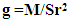(3)
with the mass of the sun as 1.99×1030 kg and radius as 6.96×108m
Using equation (3), the value of g for the sun is calculated to be approximately 274m/s2 and ﯼ as 3.65×10-3. With these, ﯼ is addressed to be the reduced magnitude of space fabric or the nature of space in the particular body, which is responsible for acceleration due to gravity, g.

#### 3.2. Example

To find the value of g for Venus using its mass and radius. [Take S= 1.50×1010m-3/kg/s2].
Putting the values of M and r for Venus from the table as 4.88× 1024kg and 6.06×106m in the equation g = M/Sr2. We have g = 4.88×1024/1.50×1010× (6.06×106)2. We have g for Venus to be approximately 8.86m/s2.
With the above calculations, we can calculate the value of g and ﯼ for any planetary body using the space constant, the mass and the radius of the particular body. i.e. using equations (3) and (2) respectively.

### 4. Results

 Table 2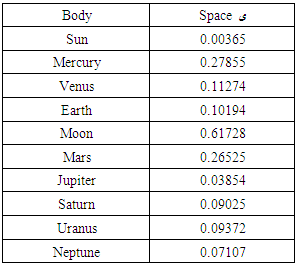Using equation , the values of ﯼ for all planetary bodies in our solar system is been calculated and concluded as shown in Table 2.
With table 2, we observed that the reduced magnitude of space fabric, ﯼ which changes from a less free space to a very free space is associated with values ranging from approximately 0.6 – 0.004.
However, we can conclude that the magnitude of space fabric is now proposed to be 1.50 × 1010m-3/kg/s2, with its subject equation as S=M/r2, where S is the space fabric.

### 5. Conclusions

#### 5.1. The Fate of the Existence of Parallel Universes

The universe is the body that encloses the outer space, and the outer space has an environment of space fabric in it, which means the outer space might just be the core of the universe, which means that there are other areas of the universe yet to be discovered. If these other areas are discovered and found to have a different environment of space [in a manner surrounding the core] other than space fabric, then it means that the enclosure consisting of all planetary bodies is a mass which is distorting a space fabric in another enclosure. Thus, our beloved enclosure will be a universe floating alone or floating next to another universe.

### ACKNOWLEDGEMENTS

I am grateful to GOD Almighty for revealing the idea used in creating the theory in this paper.

### References

  Kaku, M., (2009) Physics of the Impossible.  Gamow, G., (2013) Gravity. Courier Corporation.  Kaku, M., (2008) Parallel Worlds.  The Special and the General Theory. (2017) SPB Editors  Relativity [Uber die spezielle und die allgemeine Relativitatstheorie. Engl.]., (1920) Albert Einstein.